## Wednesday, November 5, 2014

### Pruning trees to one member per genus, and to one descendant for each clade younger than a particular age

The following request was recently posted to the R-sig-phylo email list:

“(1) I'd like to drop all but one of the tips in each genus (i.e. to generate a genus-level phylogeny). Given that all genera are monophyletic, it shouldn't matter which species I pick.

(2) I'd like to establish an era (e.g. 25 Mya) and leave only one representative of the clades there were present at that point in time.”

I submitted my replies (1, 2) but I'll repeat them here anyway.

First, let's simulate scenario (1) in which we have a species-level tree with one or more species per genus, and want to reduce the tree to a genus tree:

``````library(phytools)
## here are our species names
tips<-c("Abc_def","Abc_ghi","Def_ghi","Def_jkl",
"Ghi_jkl","Ghi_mno","Jkl_mno","Jkl_pqr")
tree<-stree(n=8,type="balanced",tip.label=tips)
plotTree(tree,ftype="i")
``````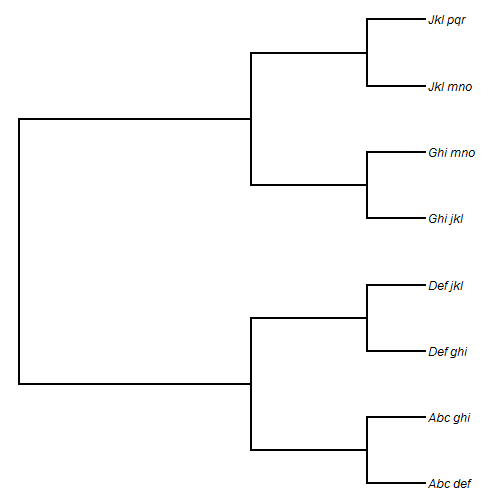Next, we'll get a list of all genus names, and prune the tree of all but one member of each genus:

``````## get a list of all genera
tips<-tree\$tip.label
genera<-unique(sapply(strsplit(tips,"_"),function(x) x))
## here are our genera
genera
``````
``````##  "Abc" "Def" "Ghi" "Jkl"
``````
``````## now drop all but one of each
ii<-sapply(genera,function(x,y) grep(x,y),y=tips)
tree<-drop.tip(tree,setdiff(tree\$tip.label,tips[ii]))
plotTree(tree,ftype="i")
``````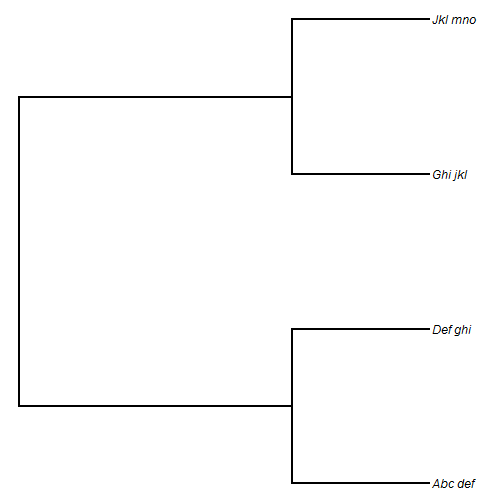Finally, we can rename our tips to be the genus names only:

``````tree\$tip.label<-sapply(strsplit(tree\$tip.label,"_"),function(x) x)
plotTree(tree,ftype="i")
``````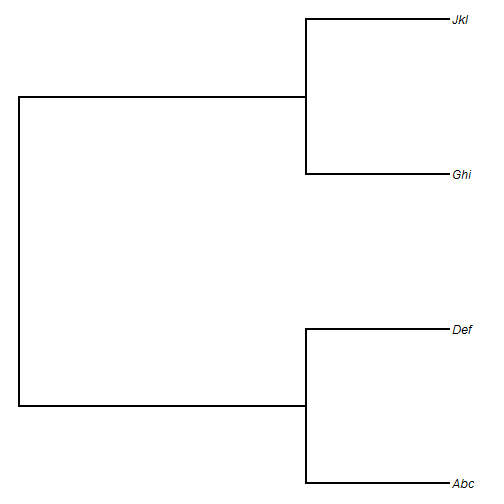That's it.

Now, let's move on to part (2). For this, I'll simulate a true tree of arbitrary total depth `100`.

``````tree<-pbtree(n=100,scale=100)
plotTree(tree,fsize=0.6)
``````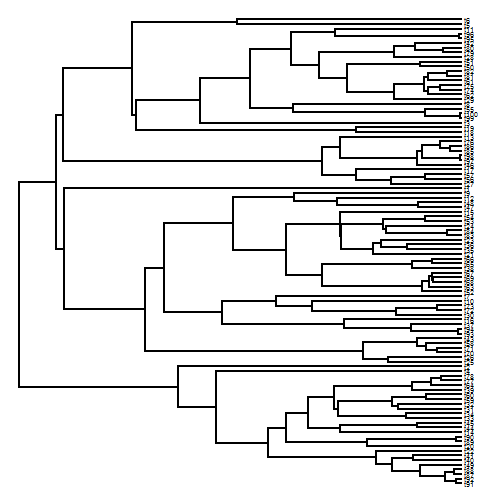Now, let's prune each clade younger than 25 units of time old to include only 1 descendant:

``````## get all node heights
H<-nodeHeights(tree)
## time from the root
t<-max(H)-25
## identify all edges that cross 25 mybp
h1<-which(H[,1]<t)
h2<-which(H[,2]>t)
ii<-intersect(h1,h2)
## all daughter nodes of those edges
nodes<-tree\$edge[ii,2]
## internal phytools function
getDescendants<-phytools:::getDescendants
## find all descendants from each edge
tips<-lapply(nodes,getDescendants,tree=tree)
## find all tips to keep
tips<-tree\$tip.label[sapply(tips,function(x,y) x[x<=Ntip(y)],y=tree)]
## drop all the others
tree<-drop.tip(tree,setdiff(tree\$tip.label,tips))
plotTree(tree)
lines(c(t,t),par()\$usr[3:4],lty="dashed",col="red")
``````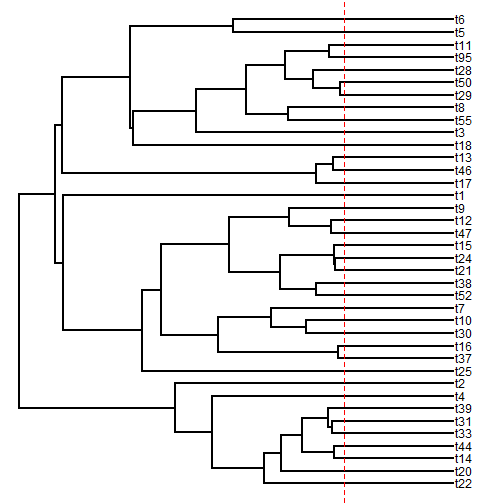Cool. It worked!

That's all for now.

1.Great post Liam, thanks!
These steps to prune trees are more or less the opposite of David Bapst's expandTaxonTree function from paleotree (in case anyone's wondering)

2.3.4.